The REG Procedure

Example 97.2 Aerobic Fitness Prediction

Aerobic fitness (measured by the ability to consume oxygen) is fit to some simple exercise tests. The goal is to develop an equation to predict fitness based on the exercise tests rather than on expensive and cumbersome oxygen consumption measurements. Three model-selection methods are used: forward selection, backward selection, and MAXR selection. Here are the data:

```*-------------------Data on Physical Fitness-------------------*
| These measurements were made on men involved in a physical   |
| fitness course at N.C.State Univ. The variables are Age      |
| (years), Weight (kg), Oxygen intake rate (ml per kg body     |
| weight per minute), time to run 1.5 miles (minutes), heart   |
| rate while resting, heart rate while running (same time      |
| Oxygen rate measured), and maximum heart rate recorded while |
| running.                                                     |
| ***Certain values of MaxPulse were changed for this analysis.|
*--------------------------------------------------------------*;
data fitness;
input Age Weight Oxygen RunTime RestPulse RunPulse MaxPulse @@;
datalines;
44 89.47 44.609 11.37 62 178 182   40 75.07 45.313 10.07 62 185 185
44 85.84 54.297  8.65 45 156 168   42 68.15 59.571  8.17 40 166 172
38 89.02 49.874  9.22 55 178 180   47 77.45 44.811 11.63 58 176 176
40 75.98 45.681 11.95 70 176 180   43 81.19 49.091 10.85 64 162 170
44 81.42 39.442 13.08 63 174 176   38 81.87 60.055  8.63 48 170 186
44 73.03 50.541 10.13 45 168 168   45 87.66 37.388 14.03 56 186 192
45 66.45 44.754 11.12 51 176 176   47 79.15 47.273 10.60 47 162 164
54 83.12 51.855 10.33 50 166 170   49 81.42 49.156  8.95 44 180 185
51 69.63 40.836 10.95 57 168 172   51 77.91 46.672 10.00 48 162 168
48 91.63 46.774 10.25 48 162 164   49 73.37 50.388 10.08 67 168 168
57 73.37 39.407 12.63 58 174 176   54 79.38 46.080 11.17 62 156 165
52 76.32 45.441  9.63 48 164 166   50 70.87 54.625  8.92 48 146 155
51 67.25 45.118 11.08 48 172 172   54 91.63 39.203 12.88 44 168 172
51 73.71 45.790 10.47 59 186 188   57 59.08 50.545  9.93 49 148 155
49 76.32 48.673  9.40 56 186 188   48 61.24 47.920 11.50 52 170 176
52 82.78 47.467 10.50 53 170 172
;
```

The following statements demonstrate the FORWARD, BACKWARD, and MAXR model selection methods:

```proc reg data=fitness;
model Oxygen=Age Weight RunTime RunPulse RestPulse MaxPulse
/ selection=forward;
model Oxygen=Age Weight RunTime RunPulse RestPulse MaxPulse
/ selection=backward;
model Oxygen=Age Weight RunTime RunPulse RestPulse MaxPulse
/ selection=maxr;
run;
```

Output 97.2.1 shows the sequence of models produced by the FORWARD model-selection method.

Output 97.2.1: Forward Selection Method: PROC REG

The REG Procedure
Model: MODEL1
Dependent Variable: Oxygen

 Forward Selection: Step 1

 Variable RunTime Entered: R-Square = 0.7434 and C(p) = 13.6988

Analysis of Variance
Source DF Sum of
Squares
Mean
Square
F Value Pr > F
Model 1 632.90010 632.90010 84.01 <.0001
Error 29 218.48144 7.53384
Corrected Total 30 851.38154

Variable Parameter
Estimate
Standard
Error
Type II SS F Value Pr > F
Intercept 82.42177 3.85530 3443.36654 457.05 <.0001
RunTime -3.31056 0.36119 632.90010 84.01 <.0001

 Bounds on condition number: 1, 1

 Forward Selection: Step 2

 Variable Age Entered: R-Square = 0.7642 and C(p) = 12.3894

Analysis of Variance
Source DF Sum of
Squares
Mean
Square
F Value Pr > F
Model 2 650.66573 325.33287 45.38 <.0001
Error 28 200.71581 7.16842
Corrected Total 30 851.38154

Variable Parameter
Estimate
Standard
Error
Type II SS F Value Pr > F
Intercept 88.46229 5.37264 1943.41071 271.11 <.0001
Age -0.15037 0.09551 17.76563 2.48 0.1267
RunTime -3.20395 0.35877 571.67751 79.75 <.0001

 Bounds on condition number: 1.0369, 4.1478

 Forward Selection: Step 3

 Variable RunPulse Entered: R-Square = 0.8111 and C(p) = 6.9596

Analysis of Variance
Source DF Sum of
Squares
Mean
Square
F Value Pr > F
Model 3 690.55086 230.18362 38.64 <.0001
Error 27 160.83069 5.95669
Corrected Total 30 851.38154

Variable Parameter
Estimate
Standard
Error
Type II SS F Value Pr > F
Intercept 111.71806 10.23509 709.69014 119.14 <.0001
Age -0.25640 0.09623 42.28867 7.10 0.0129
RunTime -2.82538 0.35828 370.43529 62.19 <.0001
RunPulse -0.13091 0.05059 39.88512 6.70 0.0154

 Bounds on condition number: 1.3548, 11.597

 Forward Selection: Step 4

 Variable MaxPulse Entered: R-Square = 0.8368 and C(p) = 4.8800

Analysis of Variance
Source DF Sum of
Squares
Mean
Square
F Value Pr > F
Model 4 712.45153 178.11288 33.33 <.0001
Error 26 138.93002 5.34346
Corrected Total 30 851.38154

Variable Parameter
Estimate
Standard
Error
Type II SS F Value Pr > F
Intercept 98.14789 11.78569 370.57373 69.35 <.0001
Age -0.19773 0.09564 22.84231 4.27 0.0488
RunTime -2.76758 0.34054 352.93570 66.05 <.0001
RunPulse -0.34811 0.11750 46.90089 8.78 0.0064
MaxPulse 0.27051 0.13362 21.90067 4.10 0.0533

 Bounds on condition number: 8.4182, 76.851

 Forward Selection: Step 5

 Variable Weight Entered: R-Square = 0.8480 and C(p) = 5.1063

Analysis of Variance
Source DF Sum of
Squares
Mean
Square
F Value Pr > F
Model 5 721.97309 144.39462 27.90 <.0001
Error 25 129.40845 5.17634
Corrected Total 30 851.38154

Variable Parameter
Estimate
Standard
Error
Type II SS F Value Pr > F
Intercept 102.20428 11.97929 376.78935 72.79 <.0001
Age -0.21962 0.09550 27.37429 5.29 0.0301
Weight -0.07230 0.05331 9.52157 1.84 0.1871
RunTime -2.68252 0.34099 320.35968 61.89 <.0001
RunPulse -0.37340 0.11714 52.59624 10.16 0.0038
MaxPulse 0.30491 0.13394 26.82640 5.18 0.0316

 Bounds on condition number: 8.7312, 104.83

The final variable available to add to the model, `RestPulse`, is not added since it does not meet the 50% (the default value of the SLE option is 0.5 for FORWARD selection) significance-level criterion for entry into the model.

The BACKWARD model-selection method begins with the full model. Output 97.2.2 shows the steps of the BACKWARD method. `RestPulse` is the first variable deleted, followed by `Weight`. No other variables are deleted from the model since the variables remaining (`Age`, `RunTime`, `RunPulse`, and `MaxPulse`) are all significant at the 10% (the default value of the SLS option is 0.1 for the BACKWARD elimination method) significance level.

Output 97.2.2: Backward Selection Method: PROC REG

 Backward Elimination: Step 0

 All Variables Entered: R-Square = 0.8487 and C(p) = 7.0000

Analysis of Variance
Source DF Sum of
Squares
Mean
Square
F Value Pr > F
Model 6 722.54361 120.42393 22.43 <.0001
Error 24 128.83794 5.36825
Corrected Total 30 851.38154

Variable Parameter
Estimate
Standard
Error
Type II SS F Value Pr > F
Intercept 102.93448 12.40326 369.72831 68.87 <.0001
Age -0.22697 0.09984 27.74577 5.17 0.0322
Weight -0.07418 0.05459 9.91059 1.85 0.1869
RunTime -2.62865 0.38456 250.82210 46.72 <.0001
RunPulse -0.36963 0.11985 51.05806 9.51 0.0051
RestPulse -0.02153 0.06605 0.57051 0.11 0.7473
MaxPulse 0.30322 0.13650 26.49142 4.93 0.0360

 Bounds on condition number: 8.7438, 137.13

 Backward Elimination: Step 1

 Variable RestPulse Removed: R-Square = 0.8480 and C(p) = 5.1063

Analysis of Variance
Source DF Sum of
Squares
Mean
Square
F Value Pr > F
Model 5 721.97309 144.39462 27.90 <.0001
Error 25 129.40845 5.17634
Corrected Total 30 851.38154

Variable Parameter
Estimate
Standard
Error
Type II SS F Value Pr > F
Intercept 102.20428 11.97929 376.78935 72.79 <.0001
Age -0.21962 0.09550 27.37429 5.29 0.0301
Weight -0.07230 0.05331 9.52157 1.84 0.1871
RunTime -2.68252 0.34099 320.35968 61.89 <.0001
RunPulse -0.37340 0.11714 52.59624 10.16 0.0038
MaxPulse 0.30491 0.13394 26.82640 5.18 0.0316

 Bounds on condition number: 8.7312, 104.83

 Backward Elimination: Step 2

 Variable Weight Removed: R-Square = 0.8368 and C(p) = 4.8800

Analysis of Variance
Source DF Sum of
Squares
Mean
Square
F Value Pr > F
Model 4 712.45153 178.11288 33.33 <.0001
Error 26 138.93002 5.34346
Corrected Total 30 851.38154

Variable Parameter
Estimate
Standard
Error
Type II SS F Value Pr > F
Intercept 98.14789 11.78569 370.57373 69.35 <.0001
Age -0.19773 0.09564 22.84231 4.27 0.0488
RunTime -2.76758 0.34054 352.93570 66.05 <.0001
RunPulse -0.34811 0.11750 46.90089 8.78 0.0064
MaxPulse 0.27051 0.13362 21.90067 4.10 0.0533

 Bounds on condition number: 8.4182, 76.851

The MAXR method tries to find the "best" one-variable model, the "best" two-variable model, and so on. Output 97.2.3 shows that the one-variable model contains `RunTime`; the two-variable model contains `RunTime` and `Age`; the three-variable model contains `RunTime`, `Age`, and `RunPulse`; the four-variable model contains `Age`, `RunTime`, `RunPulse`, and `MaxPulse`; the five-variable model contains `Age`, `Weight`, `RunTime`, `RunPulse`, and `MaxPulse`; and finally, the six-variable model contains all the variables in the MODEL statement.

Output 97.2.3: Maximum R-Square Improvement Selection Method: PROC REG

 Maximum R-Square Improvement: Step 1

 Variable RunTime Entered: R-Square = 0.7434 and C(p) = 13.6988

Analysis of Variance
Source DF Sum of
Squares
Mean
Square
F Value Pr > F
Model 1 632.90010 632.90010 84.01 <.0001
Error 29 218.48144 7.53384
Corrected Total 30 851.38154

Variable Parameter
Estimate
Standard
Error
Type II SS F Value Pr > F
Intercept 82.42177 3.85530 3443.36654 457.05 <.0001
RunTime -3.31056 0.36119 632.90010 84.01 <.0001

 Bounds on condition number: 1, 1

 The above model is the best 1-variable model found.

 Maximum R-Square Improvement: Step 2

 Variable Age Entered: R-Square = 0.7642 and C(p) = 12.3894

Analysis of Variance
Source DF Sum of
Squares
Mean
Square
F Value Pr > F
Model 2 650.66573 325.33287 45.38 <.0001
Error 28 200.71581 7.16842
Corrected Total 30 851.38154

Variable Parameter
Estimate
Standard
Error
Type II SS F Value Pr > F
Intercept 88.46229 5.37264 1943.41071 271.11 <.0001
Age -0.15037 0.09551 17.76563 2.48 0.1267
RunTime -3.20395 0.35877 571.67751 79.75 <.0001

 Bounds on condition number: 1.0369, 4.1478

 The above model is the best 2-variable model found.

 Maximum R-Square Improvement: Step 3

 Variable RunPulse Entered: R-Square = 0.8111 and C(p) = 6.9596

Analysis of Variance
Source DF Sum of
Squares
Mean
Square
F Value Pr > F
Model 3 690.55086 230.18362 38.64 <.0001
Error 27 160.83069 5.95669
Corrected Total 30 851.38154

Variable Parameter
Estimate
Standard
Error
Type II SS F Value Pr > F
Intercept 111.71806 10.23509 709.69014 119.14 <.0001
Age -0.25640 0.09623 42.28867 7.10 0.0129
RunTime -2.82538 0.35828 370.43529 62.19 <.0001
RunPulse -0.13091 0.05059 39.88512 6.70 0.0154

 Bounds on condition number: 1.3548, 11.597

 The above model is the best 3-variable model found.

 Maximum R-Square Improvement: Step 4

 Variable MaxPulse Entered: R-Square = 0.8368 and C(p) = 4.8800

Analysis of Variance
Source DF Sum of
Squares
Mean
Square
F Value Pr > F
Model 4 712.45153 178.11288 33.33 <.0001
Error 26 138.93002 5.34346
Corrected Total 30 851.38154

Variable Parameter
Estimate
Standard
Error
Type II SS F Value Pr > F
Intercept 98.14789 11.78569 370.57373 69.35 <.0001
Age -0.19773 0.09564 22.84231 4.27 0.0488
RunTime -2.76758 0.34054 352.93570 66.05 <.0001
RunPulse -0.34811 0.11750 46.90089 8.78 0.0064
MaxPulse 0.27051 0.13362 21.90067 4.10 0.0533

 Bounds on condition number: 8.4182, 76.851

 The above model is the best 4-variable model found.

 Maximum R-Square Improvement: Step 5

 Variable Weight Entered: R-Square = 0.8480 and C(p) = 5.1063

Analysis of Variance
Source DF Sum of
Squares
Mean
Square
F Value Pr > F
Model 5 721.97309 144.39462 27.90 <.0001
Error 25 129.40845 5.17634
Corrected Total 30 851.38154

Variable Parameter
Estimate
Standard
Error
Type II SS F Value Pr > F
Intercept 102.20428 11.97929 376.78935 72.79 <.0001
Age -0.21962 0.09550 27.37429 5.29 0.0301
Weight -0.07230 0.05331 9.52157 1.84 0.1871
RunTime -2.68252 0.34099 320.35968 61.89 <.0001
RunPulse -0.37340 0.11714 52.59624 10.16 0.0038
MaxPulse 0.30491 0.13394 26.82640 5.18 0.0316

 Bounds on condition number: 8.7312, 104.83

 The above model is the best 5-variable model found.

 Maximum R-Square Improvement: Step 6

 Variable RestPulse Entered: R-Square = 0.8487 and C(p) = 7.0000

Analysis of Variance
Source DF Sum of
Squares
Mean
Square
F Value Pr > F
Model 6 722.54361 120.42393 22.43 <.0001
Error 24 128.83794 5.36825
Corrected Total 30 851.38154

Variable Parameter
Estimate
Standard
Error
Type II SS F Value Pr > F
Intercept 102.93448 12.40326 369.72831 68.87 <.0001
Age -0.22697 0.09984 27.74577 5.17 0.0322
Weight -0.07418 0.05459 9.91059 1.85 0.1869
RunTime -2.62865 0.38456 250.82210 46.72 <.0001
RunPulse -0.36963 0.11985 51.05806 9.51 0.0051
RestPulse -0.02153 0.06605 0.57051 0.11 0.7473
MaxPulse 0.30322 0.13650 26.49142 4.93 0.0360

 Bounds on condition number: 8.7438, 137.13

Note that for all three of these methods, `RestPulse` contributes least to the model. In the case of forward selection, it is not added to the model. In the case of backward selection, it is the first variable to be removed from the model. In the case of MAXR selection, `RestPulse` is included only for the full model.

For the STEPWISE, BACKWARD, and FORWARD selection methods, you can control the amount of detail displayed by using the DETAILS option, and you can use ODS Graphics to produce plots that show how selection criteria progress as the selection proceeds. For example, the following statements display only the selection summary table for the FORWARD selection method (Output 97.2.4) and produce the plots shown in Output 97.2.5 and Output 97.2.6.

```ods graphics on;

proc reg data=fitness plots=(criteria sbc);
model Oxygen=Age Weight RunTime RunPulse RestPulse MaxPulse
/ selection=forward details=summary;
run;
```

Output 97.2.4: Forward Selection Summary

The REG Procedure
Model: MODEL1
Dependent Variable: Oxygen

Summary of Forward Selection
Step Variable
Entered
Number
Vars In
Partial
R-Square
Model
R-Square
C(p) F Value Pr > F
1 RunTime 1 0.7434 0.7434 13.6988 84.01 <.0001
2 Age 2 0.0209 0.7642 12.3894 2.48 0.1267
3 RunPulse 3 0.0468 0.8111 6.9596 6.70 0.0154
4 MaxPulse 4 0.0257 0.8368 4.8800 4.10 0.0533
5 Weight 5 0.0112 0.8480 5.1063 1.84 0.1871

Output 97.2.5 show how six fit criteria progress as the forward selection proceeds. The step at which each criterion achieves its best value is indicated. For example, the BIC criterion achieves its minimum value for the model at step 4. Note that this does not mean that the model at step 4 achieves the smallest BIC criterion among all possible models that use a subset of the regressors; the model at step 4 yields the smallest BIC statistic among the models at each step of the forward selection. Output 97.2.6 show the progression of the SBC statistic in its own plot. If you want to see six of the selection criteria in individual plots, you can specify the UNPACK suboption of the PLOTS=CRITERIA option in the PROC REG statement.

Output 97.2.5: Fit Criteria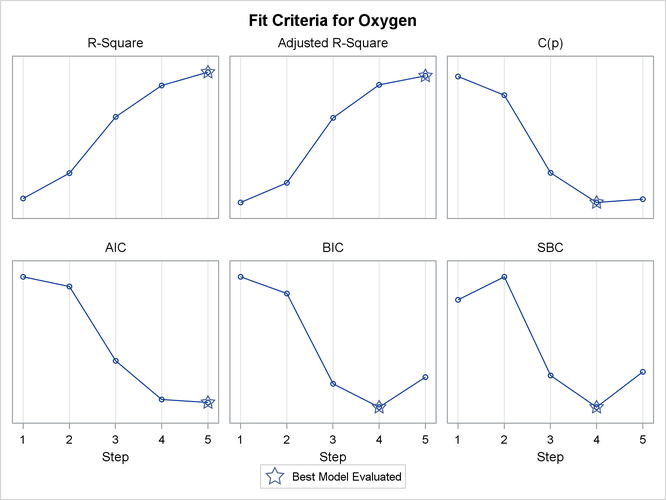Output 97.2.6: SBC Criterion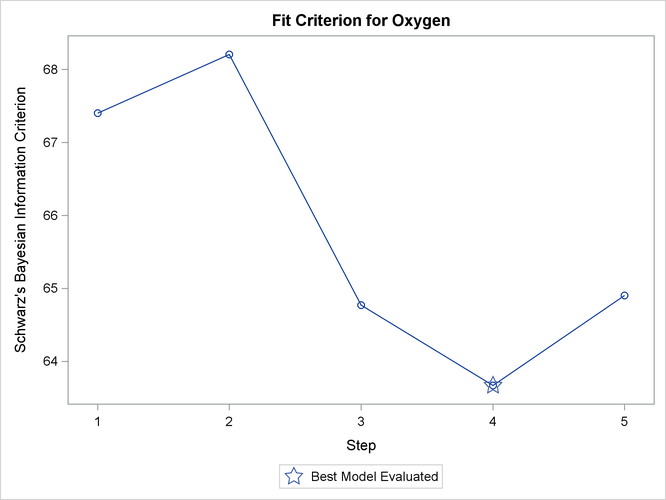Next, the RSQUARE model-selection method is used to request R square and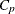statistics for all possible combinations of the six independent variables. The following statements produce Output 97.2.7:

```proc reg data=fitness plots=(criteria(label) cp);
model Oxygen=Age Weight RunTime RunPulse RestPulse MaxPulse
/ selection=rsquare cp;
title 'Physical fitness data: all models';
run;
```

Output 97.2.7: All Models by the RSQUARE Method: PROC REG

 Physical fitness data: all models

The REG Procedure
Model: MODEL1
Dependent Variable: Oxygen

R-Square Selection Method

Model
Index
Number in
Model
R-Square C(p) Variables in Model
1 1 0.7434 13.6988 RunTime
2 1 0.1595 106.3021 RestPulse
3 1 0.1584 106.4769 RunPulse
4 1 0.0928 116.8818 Age
5 1 0.0560 122.7072 MaxPulse
6 1 0.0265 127.3948 Weight
7 2 0.7642 12.3894 Age RunTime
8 2 0.7614 12.8372 RunTime RunPulse
9 2 0.7452 15.4069 RunTime MaxPulse
10 2 0.7449 15.4523 Weight RunTime
11 2 0.7435 15.6746 RunTime RestPulse
12 2 0.3760 73.9645 Age RunPulse
13 2 0.3003 85.9742 Age RestPulse
14 2 0.2894 87.6951 RunPulse MaxPulse
15 2 0.2600 92.3638 Age MaxPulse
16 2 0.2350 96.3209 RunPulse RestPulse
17 2 0.1806 104.9523 Weight RestPulse
18 2 0.1740 105.9939 RestPulse MaxPulse
19 2 0.1669 107.1332 Weight RunPulse
20 2 0.1506 109.7057 Age Weight
21 2 0.0675 122.8881 Weight MaxPulse
22 3 0.8111 6.9596 Age RunTime RunPulse
23 3 0.8100 7.1350 RunTime RunPulse MaxPulse
24 3 0.7817 11.6167 Age RunTime MaxPulse
25 3 0.7708 13.3453 Age Weight RunTime
26 3 0.7673 13.8974 Age RunTime RestPulse
27 3 0.7619 14.7619 RunTime RunPulse RestPulse
28 3 0.7618 14.7729 Weight RunTime RunPulse
29 3 0.7462 17.2588 Weight RunTime MaxPulse
30 3 0.7452 17.4060 RunTime RestPulse MaxPulse
31 3 0.7451 17.4243 Weight RunTime RestPulse
32 3 0.4666 61.5873 Age RunPulse RestPulse
33 3 0.4223 68.6250 Age RunPulse MaxPulse
34 3 0.4091 70.7102 Age Weight RunPulse
35 3 0.3900 73.7424 Age RestPulse MaxPulse
36 3 0.3568 79.0013 Age Weight RestPulse
37 3 0.3538 79.4891 RunPulse RestPulse MaxPulse
38 3 0.3208 84.7216 Weight RunPulse MaxPulse
39 3 0.2902 89.5693 Age Weight MaxPulse
40 3 0.2447 96.7952 Weight RunPulse RestPulse
41 3 0.1882 105.7430 Weight RestPulse MaxPulse
42 4 0.8368 4.8800 Age RunTime RunPulse MaxPulse
43 4 0.8165 8.1035 Age Weight RunTime RunPulse
44 4 0.8158 8.2056 Weight RunTime RunPulse MaxPulse
45 4 0.8117 8.8683 Age RunTime RunPulse RestPulse
46 4 0.8104 9.0697 RunTime RunPulse RestPulse MaxPulse
47 4 0.7862 12.9039 Age Weight RunTime MaxPulse
48 4 0.7834 13.3468 Age RunTime RestPulse MaxPulse
49 4 0.7750 14.6788 Age Weight RunTime RestPulse
50 4 0.7623 16.7058 Weight RunTime RunPulse RestPulse
51 4 0.7462 19.2550 Weight RunTime RestPulse MaxPulse
52 4 0.5034 57.7590 Age Weight RunPulse RestPulse
53 4 0.5025 57.9092 Age RunPulse RestPulse MaxPulse
54 4 0.4717 62.7830 Age Weight RunPulse MaxPulse
55 4 0.4256 70.0963 Age Weight RestPulse MaxPulse
56 4 0.3858 76.4100 Weight RunPulse RestPulse MaxPulse
57 5 0.8480 5.1063 Age Weight RunTime RunPulse MaxPulse
58 5 0.8370 6.8461 Age RunTime RunPulse RestPulse MaxPulse
59 5 0.8176 9.9348 Age Weight RunTime RunPulse RestPulse
60 5 0.8161 10.1685 Weight RunTime RunPulse RestPulse MaxPulse
61 5 0.7887 14.5111 Age Weight RunTime RestPulse MaxPulse
62 5 0.5541 51.7233 Age Weight RunPulse RestPulse MaxPulse
63 6 0.8487 7.0000 Age Weight RunTime RunPulse RestPulse MaxPulse

The models in Output 97.2.7 are arranged first by the number of variables in the model and then by the magnitude of R square for the model.

Output 97.2.8 shows the panel of fit criteria for the RSQUARE selection method. The best models (based on the R-square statistic) for each subset size are indicated on the plots. The LABEL suboption specifies that these models are labeled by the model number that appears in the summary table shown in Output 97.2.7.

Output 97.2.8: Fit Criteria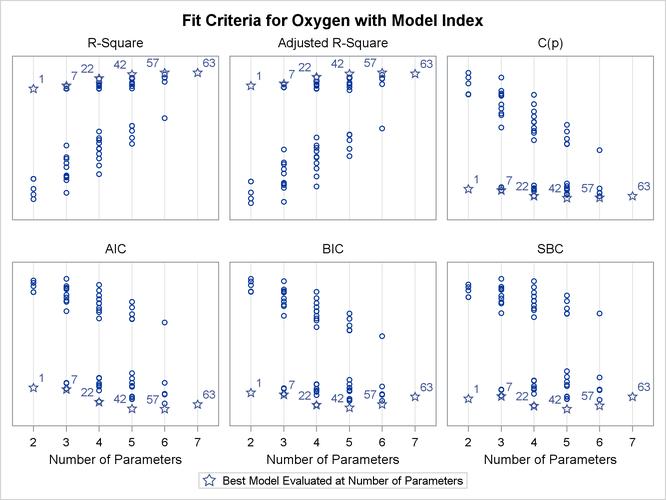Output 97.2.9 shows the plot of thecriterion by number of regressors in the model. Useful reference lines suggested by Mallows (1973) and Hocking (1976) are included on the plot. However, because all possible subset models are included on this plot, the better models are all compressed near the bottom of the plot.

Output 97.2.9:Criterion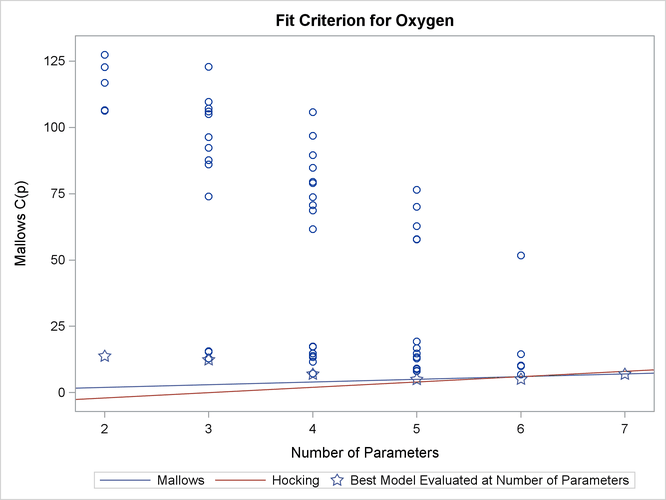The following statements use the BEST=20 option in the model statement and SELECTION=CP to restrict attention to the models that yield the 20 smallest values of thestatistic:

```proc reg data=fitness plots(only)=cp(label);
model Oxygen=Age Weight RunTime RunPulse RestPulse MaxPulse
/ selection=cp best=20;
run;

ods graphics off;
```

Output 97.2.10 shows the summary table listing the regressors in the 20 models that yield the smallestvalues, and Output 97.2.11 presents the results graphically. Reference lines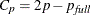and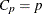are shown on this plot. See the PLOTS=CP option for interpretations of these lines. For the `Fitness` data, these lines indicate that a six-variable model is a reasonable choice for doing parameter estimation, while a five-variable model might be suitable for doing prediction.

Output 97.2.10:Selection Summary: PROC REG

The REG Procedure
Model: MODEL1
Dependent Variable: Oxygen

C(p) Selection Method

Model
Index
Number in
Model
C(p) R-Square Variables in Model
1 4 4.8800 0.8368 Age RunTime RunPulse MaxPulse
2 5 5.1063 0.8480 Age Weight RunTime RunPulse MaxPulse
3 5 6.8461 0.8370 Age RunTime RunPulse RestPulse MaxPulse
4 3 6.9596 0.8111 Age RunTime RunPulse
5 6 7.0000 0.8487 Age Weight RunTime RunPulse RestPulse MaxPulse
6 3 7.1350 0.8100 RunTime RunPulse MaxPulse
7 4 8.1035 0.8165 Age Weight RunTime RunPulse
8 4 8.2056 0.8158 Weight RunTime RunPulse MaxPulse
9 4 8.8683 0.8117 Age RunTime RunPulse RestPulse
10 4 9.0697 0.8104 RunTime RunPulse RestPulse MaxPulse
11 5 9.9348 0.8176 Age Weight RunTime RunPulse RestPulse
12 5 10.1685 0.8161 Weight RunTime RunPulse RestPulse MaxPulse
13 3 11.6167 0.7817 Age RunTime MaxPulse
14 2 12.3894 0.7642 Age RunTime
15 2 12.8372 0.7614 RunTime RunPulse
16 4 12.9039 0.7862 Age Weight RunTime MaxPulse
17 3 13.3453 0.7708 Age Weight RunTime
18 4 13.3468 0.7834 Age RunTime RestPulse MaxPulse
19 1 13.6988 0.7434 RunTime
20 3 13.8974 0.7673 Age RunTime RestPulse

Output 97.2.11:Criterion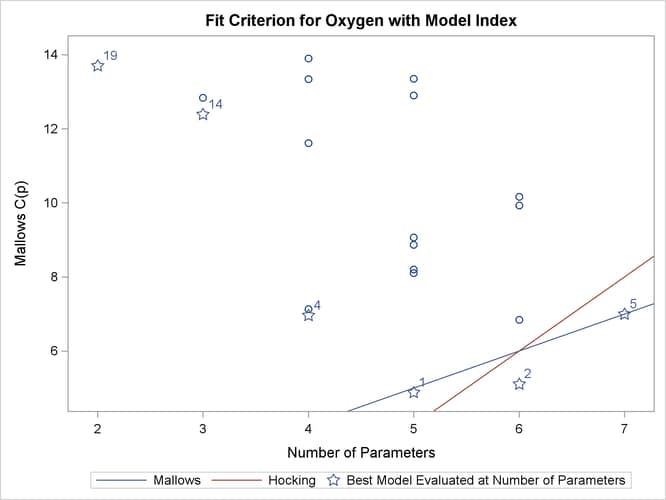Before making a final decision about which model to use, you would want to perform collinearity diagnostics. Note that, since many different models have been fit and the choice of a final model is based on R square, the statistics are biased and the p-values for the parameter estimates are not valid.